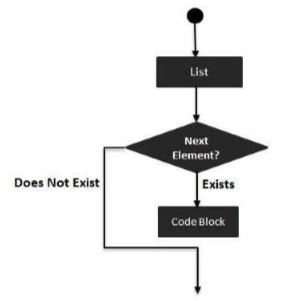# Groovy - for-in statement

The for-in statement is used to iterate through a set of values. The for-in statement is generally used in the following way.

```for(variable in range) {
statement #1
statement #2
…
}
```

The following diagram shows the diagrammatic explanation of this loop.Following is an example of a for-in statement −

```class Example {
static void main(String[] args) {
int[] array = [0,1,2,3];

for(int i in array) {
println(i);
}
}
}
```

In the above example, we are first initializing an array of integers with 4 values of 0,1,2 and 3. We are then using our for loop statement to first define a variable i which then iterates through all of the integers in the array and prints the values accordingly. The output of the above code would be −

```0
1
2
3
```

The for-in statement can also be used to loop through ranges. The following example shows how this can be accomplished.

```class Example {
static void main(String[] args) {

for(int i in 1..5) {
println(i);
}

}
}
```

In the above example, we are actually looping through a range which is defined from 1 to 5 and printing the each value in the range. The output of the above code would be −

```1
2
3
4
5
```

The for-in statement can also be used to loop through Map’s. The following example shows how this can be accomplished.

```class Example {
static void main(String[] args) {
def employee = ["Ken" : 21, "John" : 25, "Sally" : 22];

for(emp in employee) {
println(emp);
}
}
}
```

In the above example, we are actually looping through a map which has a defined set of key value entries. The output of the above code would be −

```Ken = 21
John = 25
Sally = 22
```
groovy_loops.htm